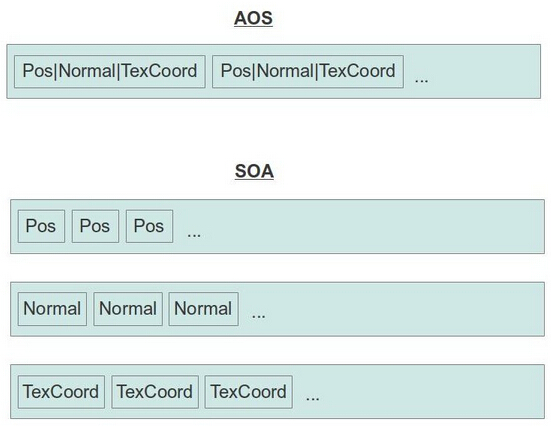modern opengl tutorial

end

# 第三十二课 顶点数组对象

## 背景## 代码

``````class Mesh
{
public:
Mesh();
~Mesh();
void Render();
private:
bool InitFromScene(const aiScene* pScene, const std::string& Filename);
void InitMesh(const aiMesh* paiMesh,
std::vector& Positions,
std::vector& Normals,
std::vector& TexCoords,
std::vector& Indices);
bool InitMaterials(const aiScene* pScene, const std::string& Filename);
void Clear();
#define INVALID_MATERIAL 0xFFFFFFFF
#define INDEX_BUFFER 0
#define POS_VB 1
#define NORMAL_VB 2
#define TEXCOORD_VB 3
GLuint m_VAO;
GLuint m_Buffers;
struct MeshEntry {
MeshEntry()
{
NumIndices = 0;
BaseVertex = 0;
BaseIndex = 0;
MaterialIndex = INVALID_MATERIAL;
}
unsigned int BaseVertex;
unsigned int BaseIndex;
unsigned int NumIndices;
unsigned int MaterialIndex;
};
std::vector m_Entries;
std::vector m_Textures;
};``````

``````bool Mesh::LoadMesh(const string& Filename)
{
// Release the previously loaded mesh (if it exists)
Clear();
// Create the VAO
glGenVertexArrays(1, &m_VAO);
glBindVertexArray(m_VAO);
// Create the buffers for the vertices atttributes
glGenBuffers(ARRAY_SIZE_IN_ELEMENTS(m_Buffers), m_Buffers);
bool Ret = false;
Assimp::Importer Importer;
const aiScene* pScene = Importer.ReadFile(Filename.c_str(), aiProcess_Triangulate |
aiProcess_GenSmoothNormals | aiProcess_FlipUVs);
if (pScene) {
Ret = InitFromScene(pScene, Filename);
}
else {
printf("Error parsing '%s': '%s'\n", Filename.c_str(), Importer.GetErrorString());
}
// Make sure the VAO is not changed from outside code
glBindVertexArray(0);
return Ret;
}``````

``````bool Mesh::InitFromScene(const aiScene* pScene, const string& Filename)
{
m_Entries.resize(pScene->mNumMeshes);
m_Textures.resize(pScene->mNumMaterials);
// Prepare vectors for vertex attributes and indices
vector Positions;
vector Normals;
vector TexCoords;
vector Indices;
unsigned int NumVertices = 0;
unsigned int NumIndices = 0;
// Count the number of vertices and indices
for (unsigned int i = 0 ; i < m_Entries.size() ; i++) {
m_Entries[i].MaterialIndex = pScene->mMeshes[i]->mMaterialIndex;
m_Entries[i].NumIndices = pScene->mMeshes[i]->mNumFaces * 3;
m_Entries[i].BaseVertex = NumVertices;
m_Entries[i].BaseIndex = NumIndices;
NumVertices += pScene->mMeshes[i]->mNumVertices;
NumIndices += m_Entries[i].BaseIndex;
}
// Reserve space in the vectors for the vertex attributes and indices
Positions.reserve(NumVertices);
Normals.reserve(NumVertices);
TexCoords.reserve(NumVertices);
Indices.reserve(NumIndices);
// Initialize the meshes in the scene one by one
for (unsigned int i = 0 ; i < m_Entries.size() ; i++) {
const aiMesh* paiMesh = pScene->mMeshes[i];
InitMesh(paiMesh, Positions, Normals, TexCoords, Indices);
}
if (!InitMaterials(pScene, Filename)) {
return false;
}
// Generate and populate the buffers with vertex attributes and the indices
glBindBuffer(GL_ARRAY_BUFFER, m_Buffers[POS_VB]);
glBufferData(GL_ARRAY_BUFFER, sizeof(Positions) * Positions.size(), &Positions,
GL_STATIC_DRAW);
glEnableVertexAttribArray(0);
glVertexAttribPointer(0, 3, GL_FLOAT, GL_FALSE, 0, 0);
glBindBuffer(GL_ARRAY_BUFFER, m_Buffers[TEXCOORD_VB]);
glBufferData(GL_ARRAY_BUFFER, sizeof(TexCoords) * TexCoords.size(), &TexCoords,
GL_STATIC_DRAW);
glEnableVertexAttribArray(1);
glVertexAttribPointer(1, 2, GL_FLOAT, GL_FALSE, 0, 0);
glBindBuffer(GL_ARRAY_BUFFER, m_Buffers[NORMAL_VB]);
glBufferData(GL_ARRAY_BUFFER, sizeof(Normals) * Normals.size(), &Normals,
GL_STATIC_DRAW);
glEnableVertexAttribArray(2);
glVertexAttribPointer(2, 3, GL_FLOAT, GL_FALSE, 0, 0);
glBindBuffer(GL_ELEMENT_ARRAY_BUFFER, m_Buffers[INDEX_BUFFER]);
glBufferData(GL_ELEMENT_ARRAY_BUFFER, sizeof(Indices) * Indices.size(), &Indices,
GL_STATIC_DRAW);
return true;
}``````

1. 使用 glBufferData() 将数据填充到缓存中；
2. 使用 glEnableVertexAttribArray() 来启用对应的顶点属性；
3. 使用 glVertexAttribPointer() 来配置顶点属性（分量的数量，分量的类型等）。

``````void Mesh::InitMesh(const aiMesh* paiMesh,
vector& Positions,
vector& Normals,
vector& TexCoords,
vector& Indices)
{
const aiVector3D Zero3D(0.0f, 0.0f, 0.0f);
// Populate the vertex attribute vectors
for (unsigned int i = 0 ; i < paiMesh->mNumVertices ; i++) {
const aiVector3D* pPos = &(paiMesh->mVertices[i]);
const aiVector3D* pNormal = &(paiMesh->mNormals[i]);
const aiVector3D* pTexCoord = paiMesh->HasTextureCoords(0) ?
&(paiMesh->mTextureCoords[i]) : &Zero3D;
Positions.push_back(Vector3f(pPos->x, pPos->y, pPos->z));
Normals.push_back(Vector3f(pNormal->x, pNormal->y, pNormal->z));
TexCoords.push_back(Vector2f(pTexCoord->x, pTexCoord->y));
}
// Populate the index buffer
for (unsigned int i = 0 ; i < paiMesh->mNumFaces ; i++) {
const aiFace& Face = paiMesh->mFaces[i];
assert(Face.mNumIndices == 3);
Indices.push_back(Face.mIndices);
Indices.push_back(Face.mIndices);
Indices.push_back(Face.mIndices);
}
}``````

``````void Mesh::Render()
{
glBindVertexArray(m_VAO);
for (unsigned int i = 0 ; i < m_Entries.size() ; i++) {
const unsigned int MaterialIndex = m_Entries[i].MaterialIndex;
assert(MaterialIndex < m_Textures.size());
if (m_Textures[MaterialIndex]) {
m_Textures[MaterialIndex]->Bind(GL_TEXTURE0);
}
glDrawElementsBaseVertex(GL_TRIANGLES,
m_Entries[i].NumIndices,
GL_UNSIGNED_INT,
(void*)(sizeof(unsigned int) * m_Entries[i].BaseIndex),
m_Entries[i].BaseVertex);
}
// Make sure the VAO is not changed from the outside
glBindVertexArray(0);
}``````

``glDeleteVertexArrays(1, &m_VAO);``